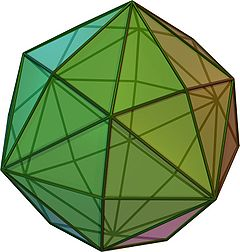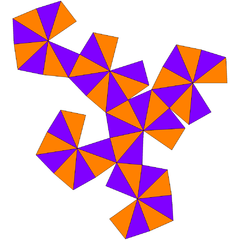# Disdyakis dodecahedron

﻿
Disdyakis dodecahedron
Disdyakis dodecahedronClick on picture for large version

Spinning version

Type Catalan
Face polygon scalene triangle
Faces 48
Edges 72
Vertices 26 = 6 + 8 + 12
Face configuration V4.6.8
Symmetry group Oh, [4,3], *432
Dihedral angle 155° 4' 56"$\arccos ( -\frac{71 + 12\sqrt{2}}{97} )$
Dual polyhedron truncated cuboctahedron
Properties convex, face-transitiveNet

In geometry, a disdyakis dodecahedron, or hexakis octahedron, is a Catalan solid and the dual to the Archimedean truncated cuboctahedron. As such it is face-transitive but with irregular face polygons. It looks a bit like an inflated rhombic dodecahedron—if one replaces each face of the rhombic dodecahedron with a single vertex and four triangles in a regular fashion one ends up with a disdyakis dodecahedron. More formally, the disdyakis dodecahedron is the Kleetope of the rhombic dodecahedron.

## Symmetry

It has Oh octahedral symmetry. Its collective edges represent the reflection planes of the symmetry.

## Dimensions

If its smallest edges have length 1, its surface area is$\frac{6}{7}\sqrt{783+436\sqrt{2}}$ and its volume is$\frac{1}{7}\sqrt{3(2194+1513\sqrt{2})}$.